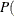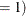### Empirical Power Simulation (DATA Step, SAS/STAT Software)

You can obtain a highly accurate power estimate by simulating the power empirically. You need to use this approach for analyses that are not supported directly in SAS/STAT tools and for which you lack a power formula. But the simulation approach is also a viable alternative to existing power approximations. A high number of simulations will yield a more accurate estimate than a non-exact power approximation.

Although exact power computations for the two-sample t test are supported in several of the SAS/STAT tools, suppose for purposes of illustration that you want to simulate power for the continuing t test example. This section describes how you can use the DATA step and SAS/STAT software to do this.

The simulation involves generating a large number of data sets according to the distributions defined by the power analysis input parameters, computing the relevant p-value for each data set, and then estimating the power as the proportion of times that the p-value is significant.

The following statements compute a power estimate along with a 95% confidence interval for power for the first scenario in the two-sample t test example, with 10,000 simulations:

```%let meandiff =     5;
%let stddev   =    12;
%let alpha    =  0.05;
%let ntotal   =   100;
%let nsim     = 10000;

data simdata;
call streaminit(123);
do isim = 1 to &nsim;
do i = 1 to floor(&ntotal/2);
group = 1;
y = rand('normal', 0        , &stddev);
output;
group = 2;
y = rand('normal', &meandiff, &stddev);
output;
end;
end;
run;

ods listing close;
proc ttest data=simdata;
ods output ttests=tests;
by isim;
class group;
var y;
run;
ods listing;

data tests;
set tests;
where method="Pooled";
issig = probt < &alpha;
run;
```
```proc freq data=tests;
ods select binomialprop;
tables issig / binomial(level='1');
run;
```

First the DATA step is used to randomly generate nsim = 10,000 data sets based on the meandiff, stddev, and ntotal parameters and the normal distribution, consistent with the assumptions underlying the two-sample t test. These data sets are contained in a large SAS data set called `simdata` indexed by the variable `isim`.

The CALL STREAMINIT(123) statement initializes the random number generator with a specific sequence and ensures repeatable results for purposes of this example. ( Note: Skip this step when you are performing actual power simulations.)

The TTEST procedure is run using `isim` as a BY variable, with the ODS LISTING CLOSE statement to suppress output. The ODS OUTPUT statement saves the TTests table to a data set called `tests`. The p-values are contained in a column called probt.

The subsequent DATA step defines a variable called `issig` to flag the significant p-values.

Finally, the FREQ procedure computes the empirical power estimate as the estimate of`issig`and provides approximate and exact confidence intervals for this estimate.

Figure 18.7 shows the results. The estimated power is 0.5388 with 95% confidence interval (0.5290, 0.5486). Note that the exact power of 0.541 shown in the first row in Figure 18.1 is contained within this tight confidence interval.

Figure 18.7: Simulated Power (DATA Step, SAS/STAT Software)

The FREQ Procedure

Binomial Proportion
issig = 1
Proportion 0.5388
ASE 0.0050
95% Lower Conf Limit 0.5290
95% Upper Conf Limit 0.5486

Exact Conf Limits
95% Lower Conf Limit 0.5290
95% Upper Conf Limit 0.5486